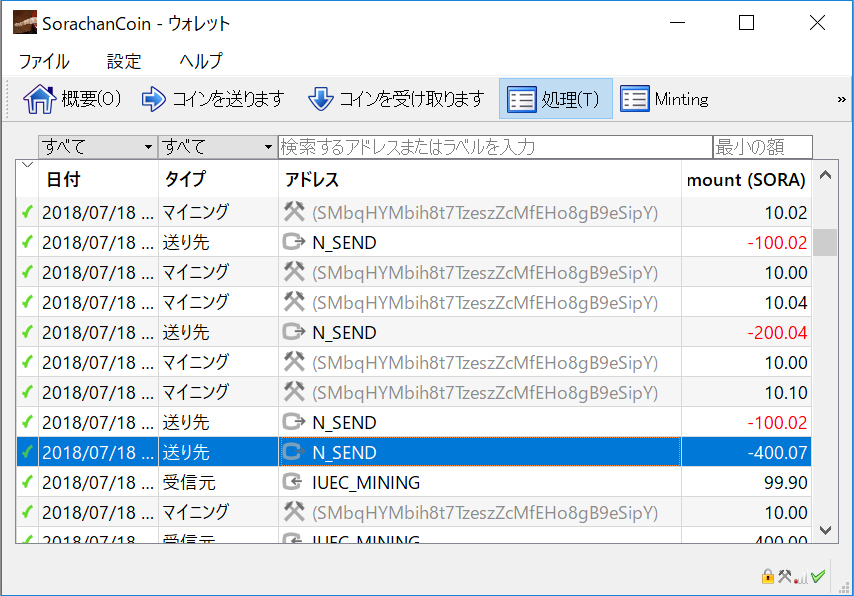# SORA project

SORA project

Bitcointalk: > here

#### Introduction

Quantum resistance has become a topic of discussion, but I have become skeptical about whether it is an implementation that needs to be immediately applied to blockchain.

Certainly, due to Shor's algorithm, problems arise concerning RSA, However, blockchain does not depend on the RSA mechanism.

While it does grapple with the common issues of the discrete logarithm problem, the usage and effects differ, so the problems with RSA are not the problems of the blockchain.

In reality, it seems that the current system might be sufficient for about another 30 years. Surprisingly, the situation might resolve in this system.

#### Quantum Computers and Shor's Algorithm

Quantum computers process information using quantum bits (qubits). This allows them to perform calculations much faster than classical computers. Shor's algorithm is one of the algorithms executed on quantum computers, efficiently factoring large numbers.

$| \psi \rangle = \cos\left(\frac{\theta}{2}\right) | 0 \rangle + e^{i\phi} \sin\left(\frac{\theta}{2}\right) | 1 \rangle$

Modern public key cryptography relies on the fact that factoring large numbers is not computationally feasible in a realistic timeframe. However, quantum computers can factor large numbers in a realistic timeframe using Shor's algorithm.

Originally, the phase information φ of a quantum bit in superposition cannot be directly observed. However, by transforming this phase information into the frequency domain through quantum Fourier transform, it can be converted into an observable probability amplitude. And this frequency domain problem also affects the discrete logarithm problem faced by elliptic curves.

$f(x) = a^x \mod N, \;\gcd(a, N) = 1$ $|x\rangle = H\; \otimes \;H\; \otimes \cdots \otimes \;H \;|00 \cdots 0\rangle = \sum_k |k\rangle \rightarrow f(x) \rightarrow |x\rangle\; \otimes \;|f(x)\rangle$ $|f(x)\rangle = \sum_k |k\rangle \rightarrow \text{Collapse} \rightarrow |f(x)\rangle \rightarrow f(j),\ j: \text{the chosen solution}$ $\therefore\;|j\rangle = \frac{1}{\sqrt{n}} ( |m_1\rangle + |m_2\rangle + \ldots + |m_n\rangle )$ $\text{n: the number of superposed states on the input register}$ $m_1 \cdots m_n: \text{the respective states}$

$\therefore\;QFT\_{2^n}\;|j\rangle \rightarrow \frac{1}{\sqrt{2^n}} \sum_{k=0}^{2^n - 1} e^{2\pi i j k / 2^n} |k\rangle$

#### SORA project dedicates to quantum computing, therefore, the development of features has been inherited as follows link. *** Welcome to SORA Neural Network, the "FromHDDtoSSD v3" *** ＞＞ https://www.fromhddtossd.com/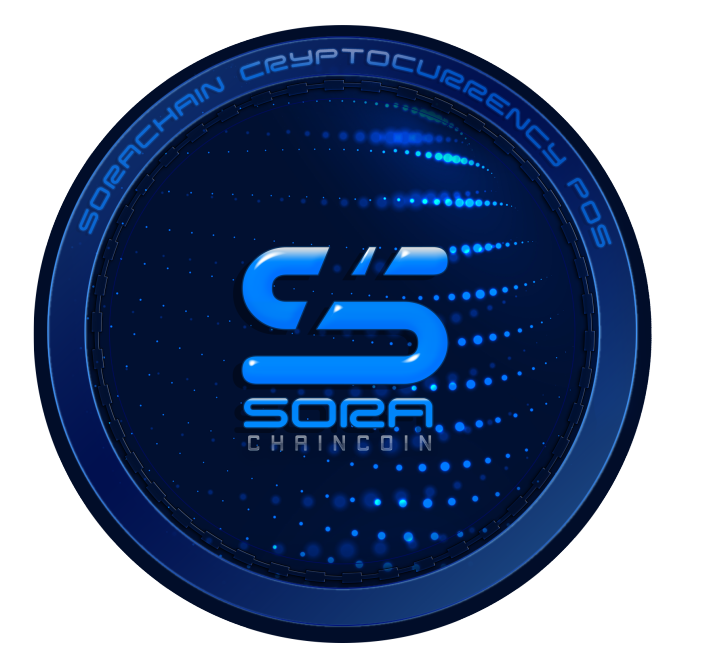## B, About SORA implements transactions, Smart contracts, AI-NFTs, Quantum Resistant Key.

#### AI-NFT

Support for ownership management, metaverse, drive(HDD/SSD/NVMe) inspection and advanced scientific analysis ... etc. Web3 - Blockchain - Multidimensional NFT by SORA Network. We aim to popularize multidimensional NFTs that can be built by direct product based on Web3 - blockchain technology. The base development has already been completed, and 1-dim NFT, 2-dim NFT, and 4-dim NFT are operating normally on SORA Network.

What kind of use is there? For example, when analyzing meteorological observation data with a blockchain. If the "wind direction" and "wind strength" at a certain point are used as data, it will be a 2-dim NFT. This 2-dim NFT operate to record data on the SORA-blockchain. And if you give a special NFT to blockchain with action by direct product or comparison, you can get the result from the blockchain. By the way, regarding the first goal of the SORA project, "Achieving drive inspection statistics on the blockchain", we achieved with the 4-dim NFT (i-sector). Now it can operate SSD/NVMe safely.

$a_0 \cdots a_n \in \text{AI-NFT}$ $T_{ij} = a_0 \otimes a_1 \otimes a_2 \otimes \cdots \otimes a_n$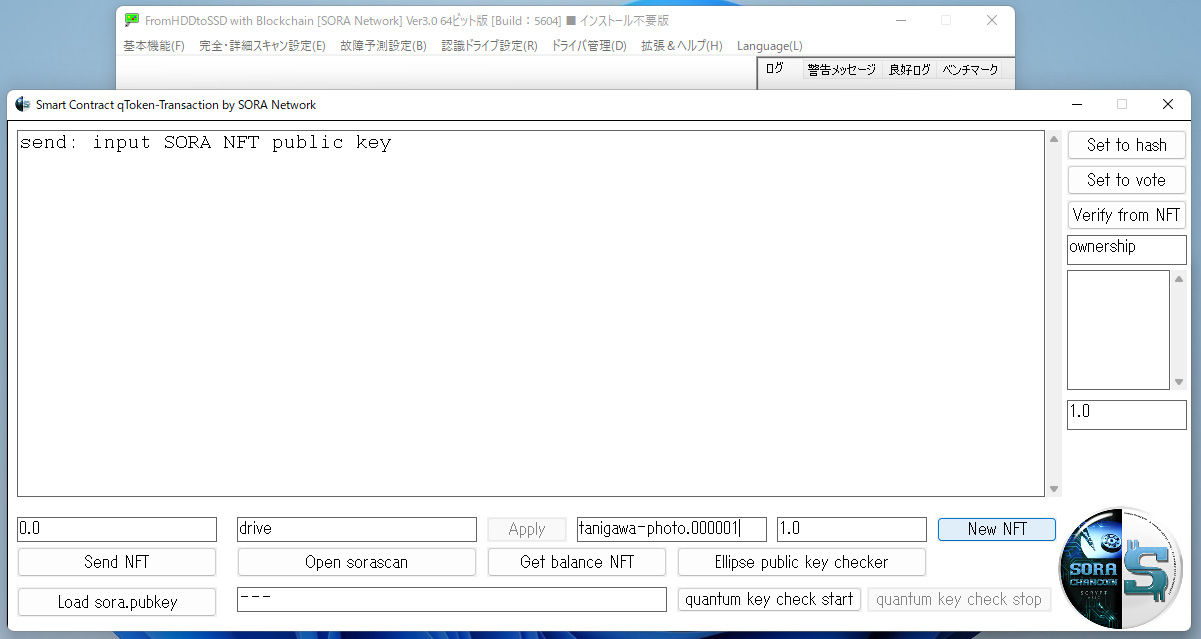#### 16 passphrase, restore wallet completely

With this kind of feeling, you can build an NFT that can directly product the conventional tokens with smart contract and raise the dimension. Smartly adapts to any application ... Reborn as a general blockchain! Then, No need a hard-ware wallet, No need a paper wallet, No need a buckup, in SORA Network. No need, those backup, hardware wallet, paper wallet, and so on. Why? because Using 16 passphrase, restore wallet completely (Coins and NFTs are safe).

$f: D^{16} \longrightarrow C$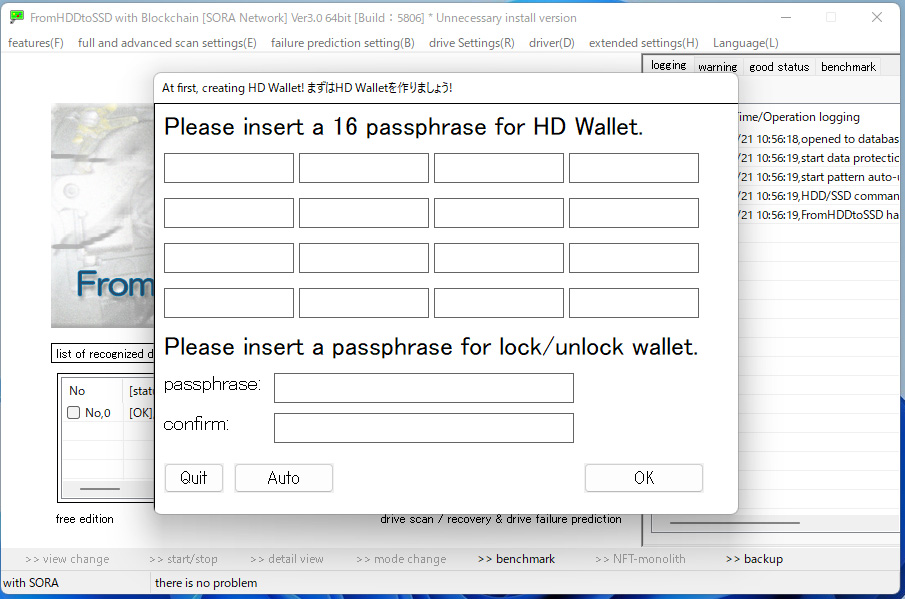## C, SORA Neural Network Web3.0 blockchain: SORA Neural Network

### The Dragons cave cryptocurrency community https://www.thedragonscave.cc/crypto/

In this cryptocurrency community, we deal with topics such as blockchain quantum resistance.## D, Exchanges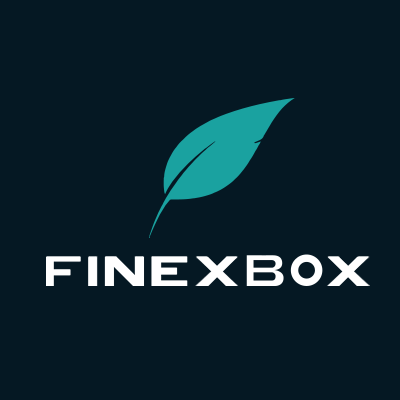### SORA/BTC https://www.finexbox.com/market/pair/SORA-BTC.html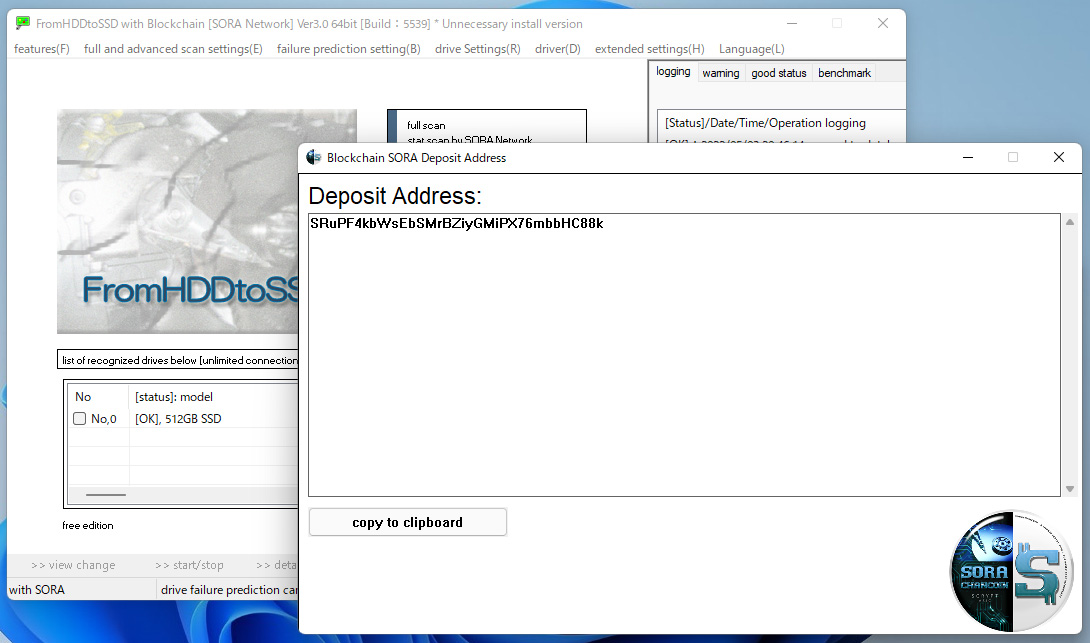### SORA project dedicates to quantum computing, therefore, the development of features has been inherited as follows link. [Welcome to SORA Neural Network, the "FromHDDtoSSD v3"] https://www.fromhddtossd.com/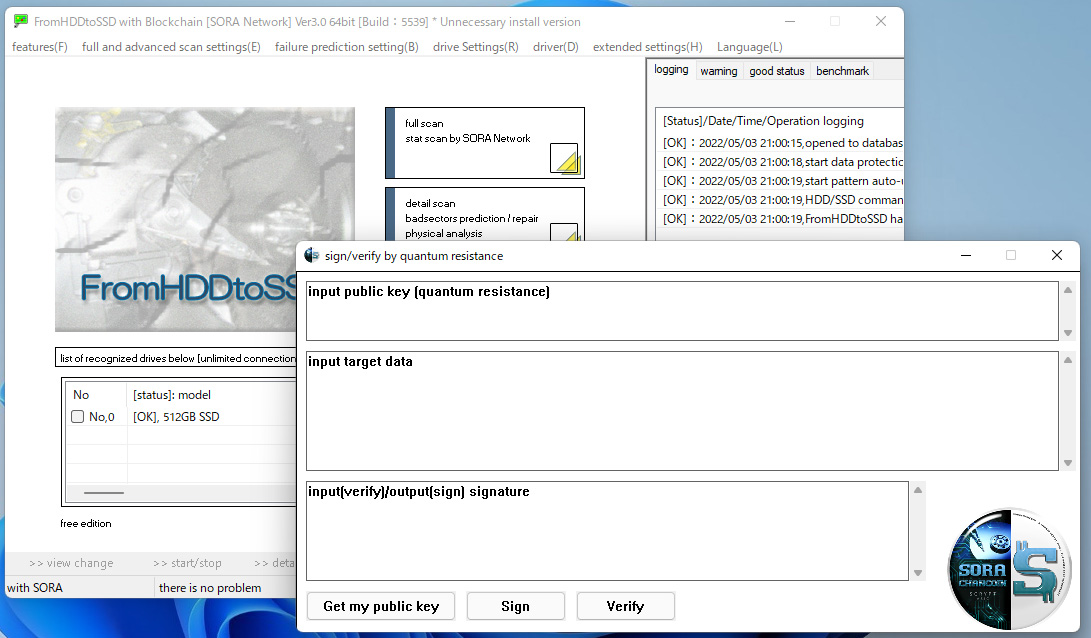#### We implemented latest SQLite, and using wallet database, replace BerkeleyDB to SQLite.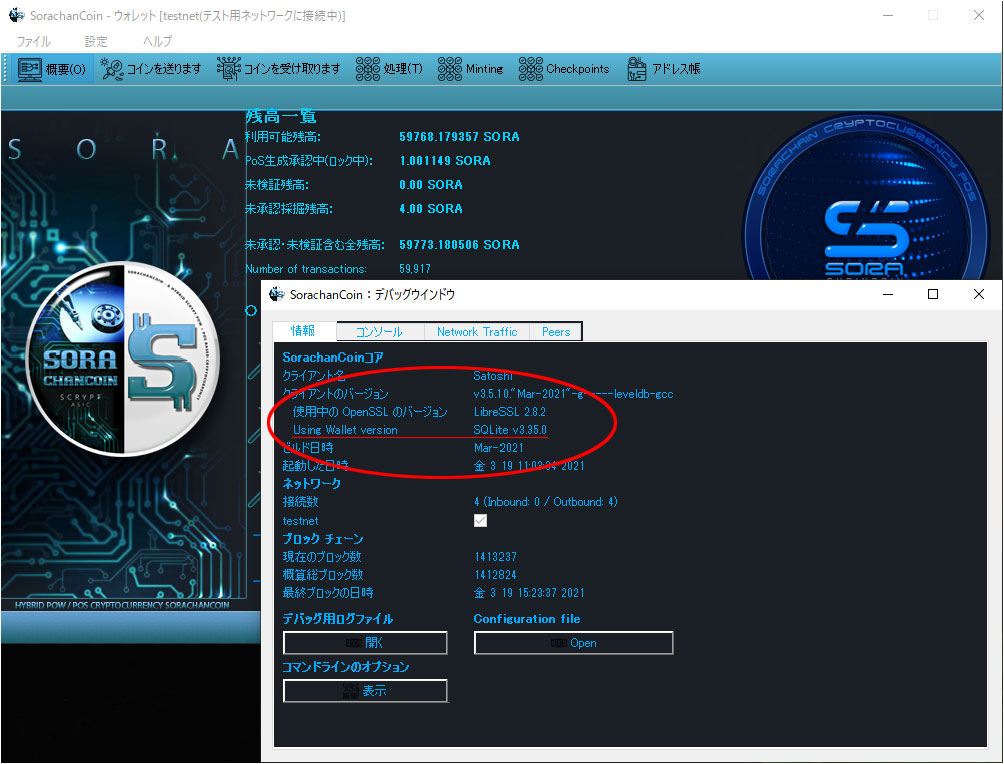#### We have implemented automatic checkpoints.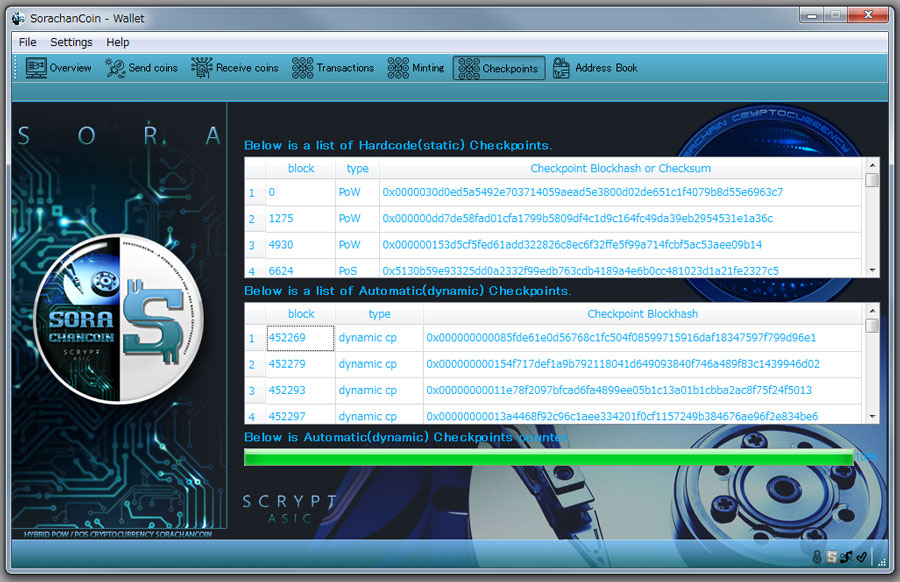#### We implemented peers tab.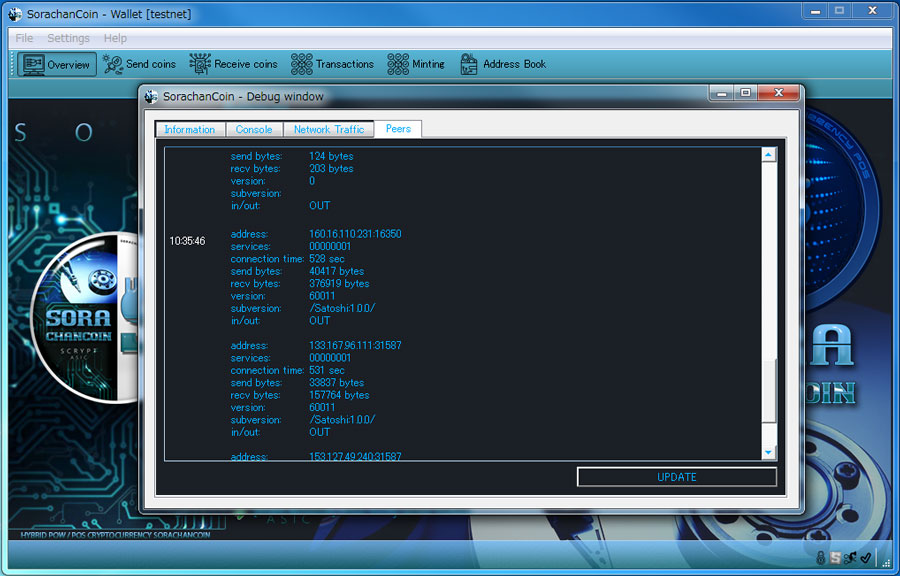#### We released the "SorachanCoin Wallet with a benchmark".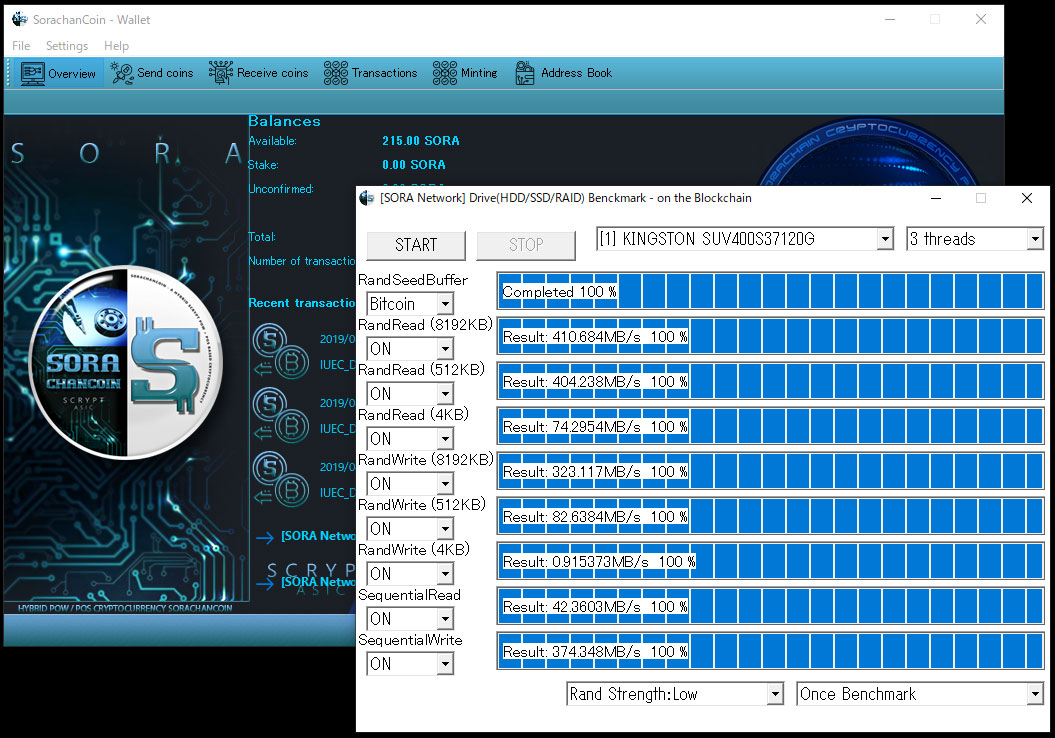#### We launched SorachanCoin project, SorachanCoin-qt ver1.0.